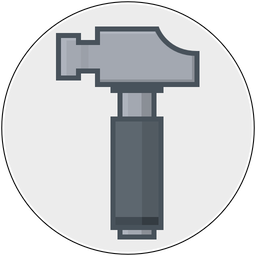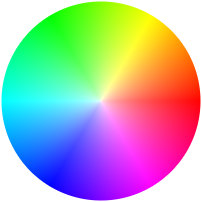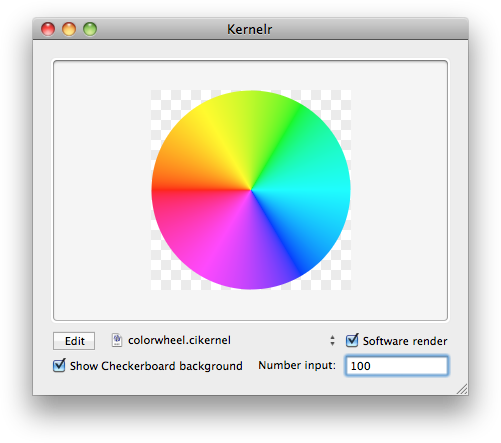The Shape of Everything
A website mostly about Mac stuff, written by August "Gus" Mueller
» Acorn
» Retrobatch
» Mastodon
» Micro.blog
» Instagram
» Github
» Maybe Pizza?
» Archives
» Feed
» Micro feed
July 29, 2010

For some reason recently, I wanted to see if I could write a Core Image filter to make a color wheel. Ok, that's kind of a lie. I recently came across Apple's private CIColorWheelGenerator filter and I was really intrigued at how they did it. Here's what the output from it looks like:(Exhibit A, the output from Apple's private color wheel generator)

I didn't spend much time on it, but my friend George Codillo did, and here's what he came up with (along with some help from Andy Finnell's HSB mixer CI Kernel).(Exhibit B, the color wheel in Acorn's Kernelr sample code, as coded up by George.)

And here's his code:

```bool isOdd(int v) {
float dividend = float(v) / 2.0;
return dividend != floor(dividend);
}

vec4 hsvToRgb(vec4 hsv) {
float h = hsv.r;
float s = hsv.g;
float v = hsv.b;
int i = int(floor(h));
float f = isOdd(i) ? h - float(i) : 1.0 - (h - float(i));
float m = v * (1.0 - s);
float n = v * (1.0 - s * f);
vec4 result = (i == 0) ? vec4(v, n, m, hsv.a) : ((i == 1) ?
vec4(n, v, m, hsv.a) : ((i == 2) ? vec4(m, v, n, hsv.a) : ((i == 3) ?
vec4(m, n, v, hsv.a) : ((i == 4) ? vec4(n, m, v, hsv.a) : ((i == 5) ?
vec4(v, m, n, hsv.a) : vec4(v, n, m, hsv.a))))));

return (h == -1.0) ? vec4(v, v, v, hsv.a) : result;
}

kernel vec4 colorWheel(sampler src, float radius) {

vec2 v0 = destCoord();
float a = distance(v0, v1);
float b = distance(v1, center);

float angle = atan(a,b);

float pi = 3.14159265;
(atan(b,a)+pi/2.0):atan(a,b)+pi):

vec4 theColor = vec4(1.0);

theColor.r = (angle * 3.0) / pi;

// make it a cirlce
float dist = length(destCoord() - center);
theColor.a = clamp(radius - dist, 0.0, 1.0);

return premultiply(hsvToRgb(theColor));
}```

(You can also get away with coding this up in Quartz Composer, but I've found it to be a bit of a pain. Rather, you should check out Kernelr which is an Acorn plugin for testing CI Kernels and is available as part of the Acorn SDK.)

Got another implementation to share? Let me know and I'll link to it!

Update: Jens Ayton points to a GLSL implementation: http://gist.github.com/499357

Math is neat.

I'm on a Boat.